# IB DP Physics: HL复习笔记7.3.4 Particle Conservation Laws

### Particle Conservation Laws

#### Charge

• Electric charge must be conserved during particle interactions
• This means charge must have the same value overall before and after the interaction
• Charge is found in quarks, leptons and exchange particles and their anti-matter counterparts

#### Baryon Number

• The baryon number, B, is the number of baryons in an interaction
• B depends on whether the particle is a baryon, anti-baryon or neither
• Baryons have a baryon number B = +1
• Anti-baryons have a baryon number B = –1
• Particles that are not baryons have a baryon number B = 0
• Baryon number is a quantum number and is conserved in all interactions
• This is one of the indicators for whether an interaction is able to occur or not

#### Lepton number

• The lepton number, L, is the number of leptons in an interaction
• L depends on whether the particle is a lepton, anti-lepton or neither
• Leptons have a lepton number L = +1
• Anti-leptons have a lepton number L = –1
• Particles that are not leptons have a lepton number L = 0
• Lepton number is a quantum number and is conserved in all interactions
• This is one of the indicators for whether an interaction is able to occur or not

#### Conservation Laws

• All particle interactions must obey a set of conservation laws. These are conservation of:
• Charge, Q
• Baryon number, B
• Lepton Number, L
• Strangeness, S
• Energy (or mass-energy)
• Momentum
• However, strangeness does not need to be conserved in weak interactions. It can change by either 0, +1 or –1
• Quantum numbers such as QB, L and S can only take discrete values (ie. 0, +1, –1, 1/2)
• To know whether a particle interaction can occur, check whether each quantum number is equal on both sides of the equation
• If even one of them, apart from strangeness in weak interactions, is not conserved then the interaction cannot occur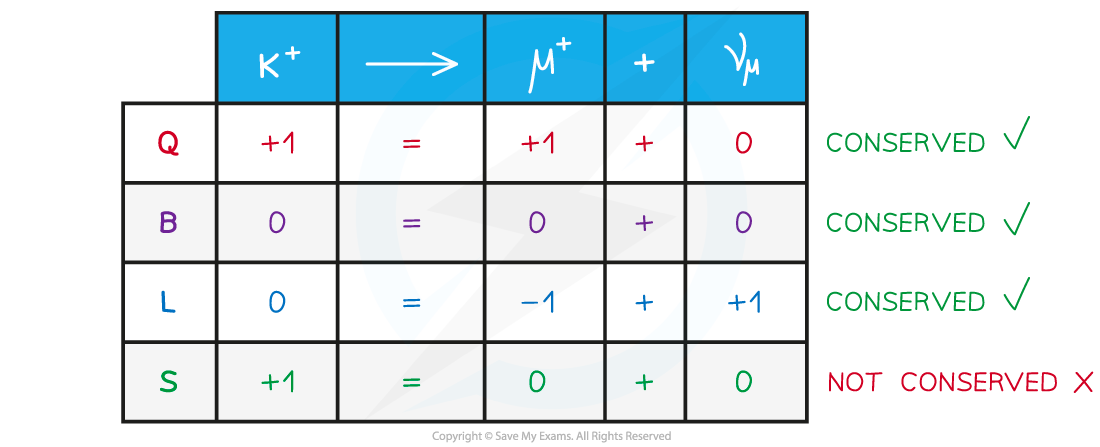Example of a working out what is conserved in Kaon decay. This decay must be through the weak interaction since S is not conserved

#### Strangeness

• Strangeness, S, like baryon and lepton number, is a quantum number
• Strangeness is conserved in every interaction except the weak interaction
• This means that strange particles are always produced in pairs (e.g. K+ and K–)
• S depends on whether the particle contains a strange quark, anti-strange quark, or no strange quarks
• Particles with an anti-strange quark have S = +1
• Particle with a strange quark have S = –1
• Particles with no strange quark have S = 0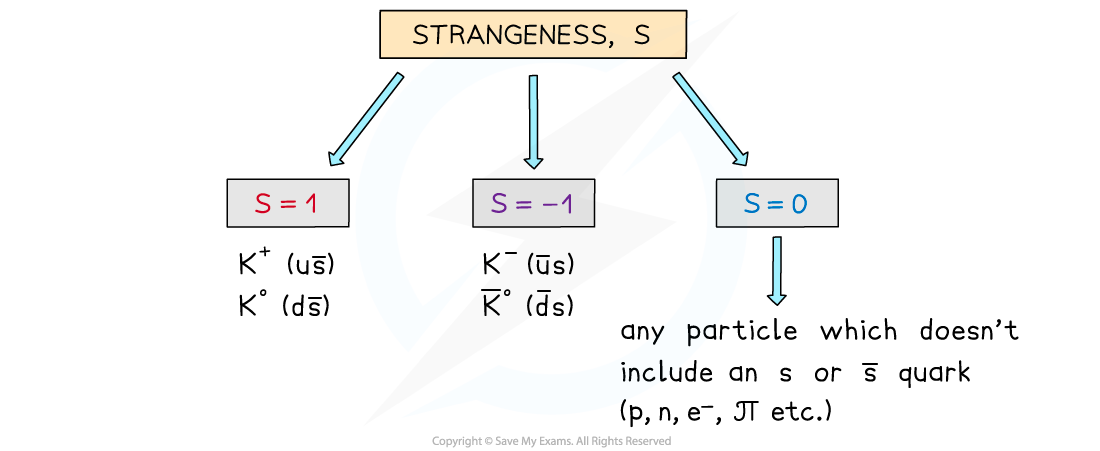Only particles with a strange or anti-strange quark have a strangeness of +1 or –1

• Strangeness can change by 0, +1 or –1 in weak interactions

#### Strange Particles

• Strange particles are particles that include a strange or anti-strange quark
• An example of these are kaons
• Strange particles always:
• Are produced through the strong interaction
• Decay through the weak interaction
• An example of a kaon production could be: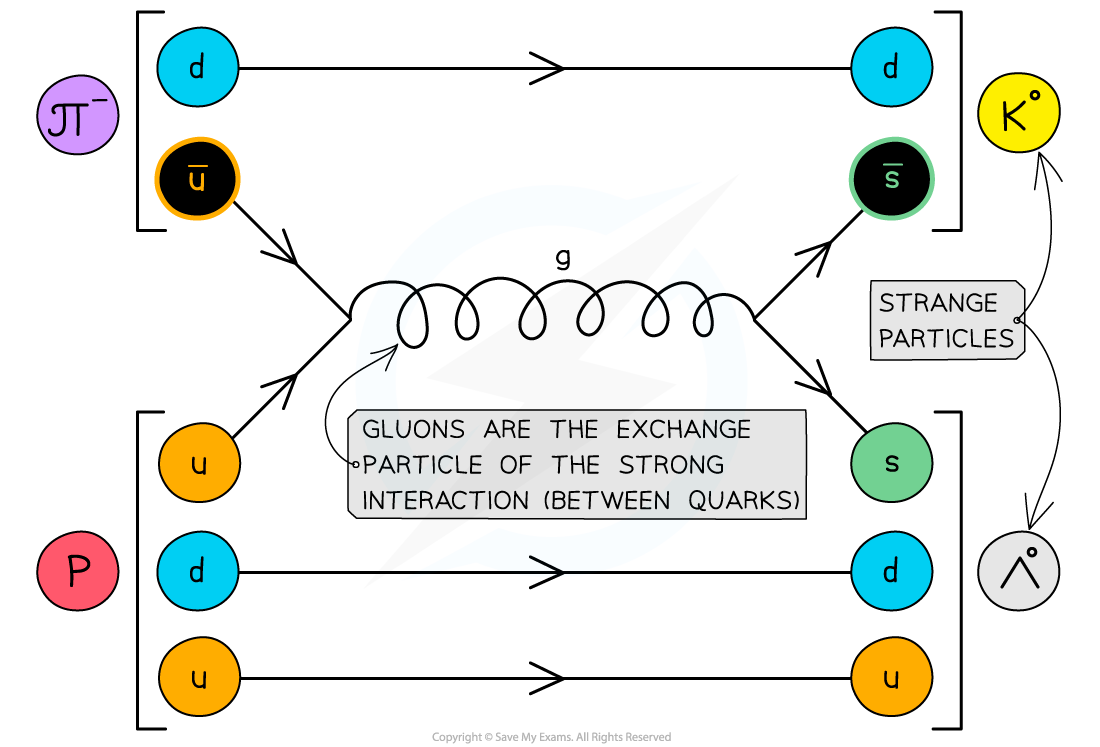Kaons are produced through the strong interaction. This is shown by the gluon exchange particle.

• An example of kaon decay could be: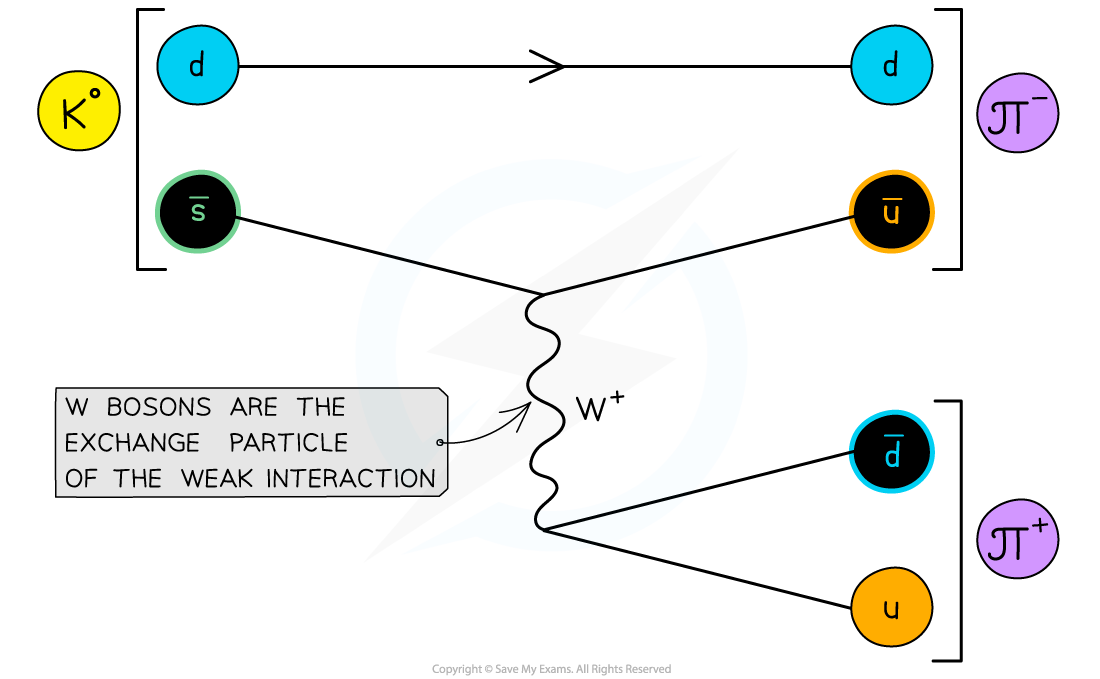Kaons decay via the weak interaction. This is shown by the W+ boson.

#### Worked Example

The lambda nought particle Λ0 is has a quark composition uds.

Show, in terms of the conservation of charge, strangeness, baryon number and lepton number whether the following interaction is permitted:Step 1: Determine conservation of charge, Q

• The Λ0 has a charge of 0
• The pion π has a charge of –1
• The positron e+ has a charge of +1
• The electron neutrino νe has a charge of 0

0 = –1 + 1 + 0

• Therefore, charge is conserved

Step 2: Determine conservation of strangeness, S

• Λ0 has an s quark, so must have a strangeness of –1
• None of the particles on the right hand side of the decay has a strange quark

–1 = 0 + 0 + 0

• Therefore, strangeness is not conserved

Step 3: Determine conservation of baryon number, B

• Λ0 is a baryon since it has 3 quarks, so must have a baryon number of +1
• The pion π is a meson so has a baryon number 0
• The positron is a lepton so has a baryon number 0
• The electron neutrino νe is a lepton so has a baryon number 0

+1 = 0 + 0 + 0

• Therefore, baryon number is not conserved

Step 4: Determine conservation of lepton number, L

• Λ0 is a baryon, so must have a lepton number of 0
• The pion π is a meson so has a lepton number of 0
• The positron e+ is an anti-lepton so has a lepton number of –1
• The electron neutrino νe is a lepton so has a lepton number of +1

0 = 0 + (–1) + 1

• Therefore, lepton number is conserved

Step 5: Conclusion

• Since the baryon number is not conserved, this interaction is not permitted

#### Worked Example

The equation for β decay is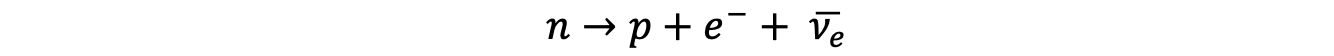Using the quark model of beta decay, prove that the charge is conserved in this decay.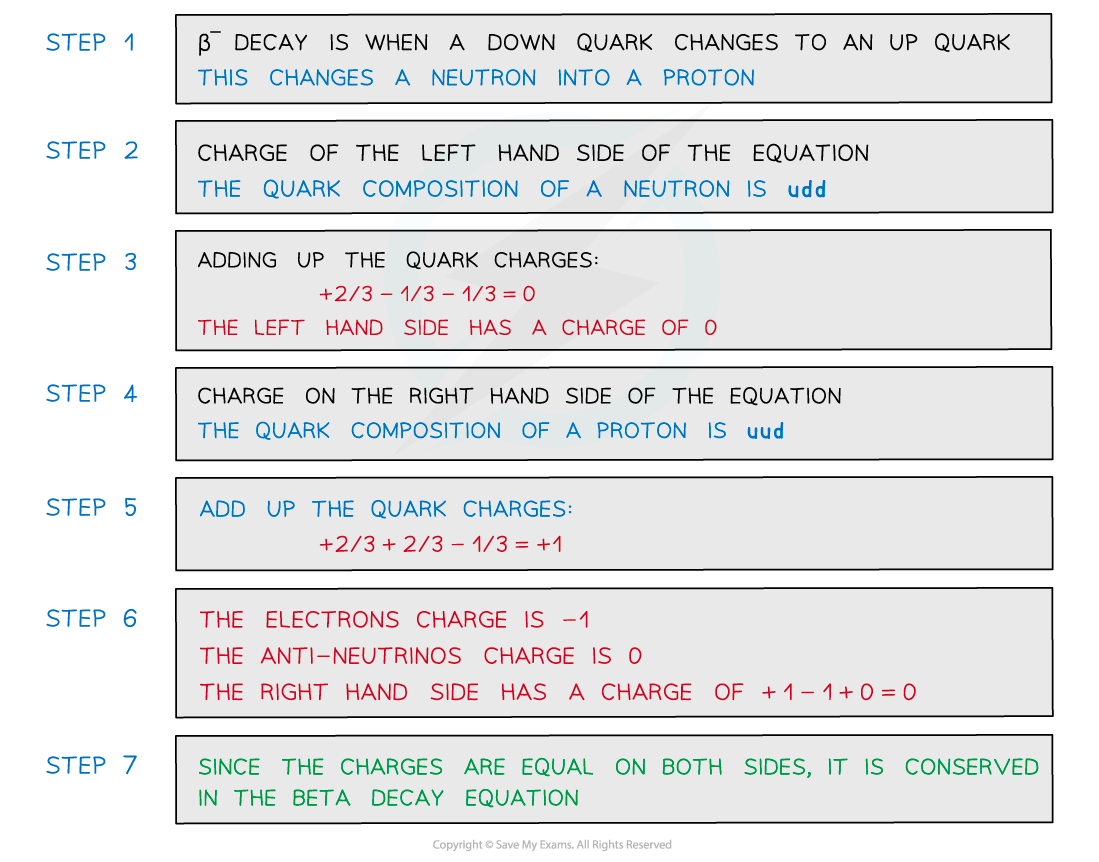#### Worked Example

The sigma baryon has a quark structure of suu. It decays to produce a proton and pion as shown in the equation belowProve that this decay is via the weak interaction.

Step 1: Determine the strangeness, S of each particle

• Since sigma baryon has one s quark, it has S = –1
• The proton and pion has no strange particles, so they have S = 0

Step 2: Determine strangeness, S on both sides of the equation

• The sigma baryon has a S = –1 but the meson and proton have a S = 0

–1 = 0 + 0

Step 3: Comment on the conservation of strangeness

• Since S is not conserved on both sides of the decay equation (only changed by –1), this decay is via the weak interaction
• This is because S is conserved in all other types of interaction (strong and EM), but isn't always conserved in weak interactions